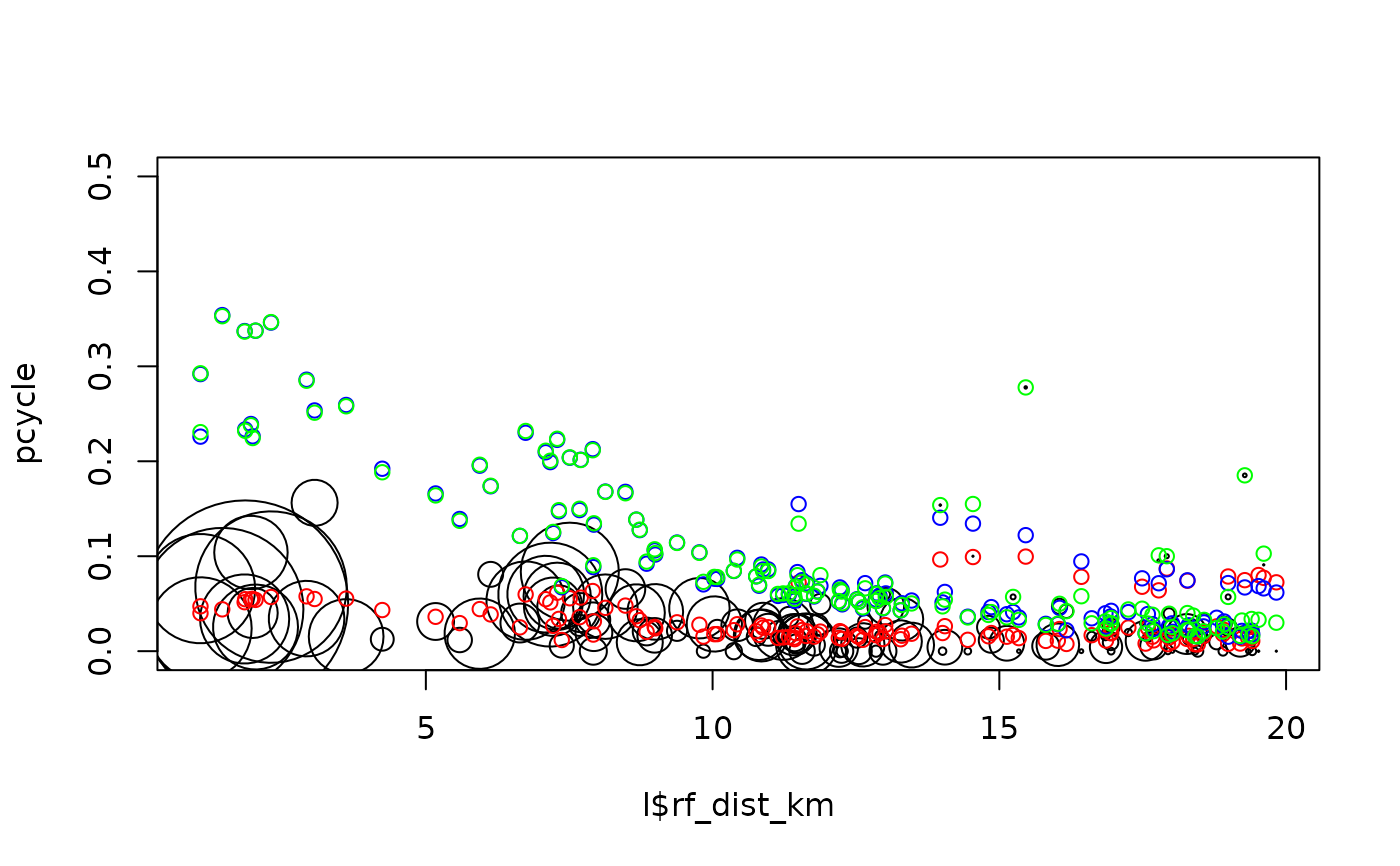Model cycling levels as a function of explanatory variables

model_pcycle_pct_2020(pcycle, distance, gradient, weights)

## Arguments

pcycle The proportion of trips by bike, e.g. 0.1, meaning 10% Vector distance numeric values of routes in km (switches to km if more than 100). Vector gradient numeric values of routes. The weights used in the model, typically the total number of people per OD pair

## Examples

# l = get_pct_lines(region = "isle-of-wight")
# l = get_pct_lines(region = "cambridgeshire")
l = wight_lines_pct
pcycle = l$bicycle / l$all
pcycle_dutch = l$dutch_slc / l$all
m1 = model_pcycle_pct_2020(
pcycle,
distance = l$rf_dist_km, gradient = l$rf_avslope_perc - 0.78,
weights = l$all ) m2 = model_pcycle_pct_2020( pcycle_dutch, distance = l$rf_dist_km,
gradient = l$rf_avslope_perc - 0.78, weights = l$all
)
m3 = model_pcycle_pct_2020(
pcycle_dutch, distance = l$rf_dist_km, gradient = l$rf_avslope_perc - 0.78,
weights = rep(1, nrow(l))
)
m1
#>
#> Call:  stats::glm(formula = pcycle ~ distance + sqrt(distance) + I(distance^2) +
#>     family = "quasibinomial", weights = weights)
#>
#> Coefficients:
#>             (Intercept)                 distance           sqrt(distance)
#>                -6.79130                 -1.04186                  4.17349
#>                 0.01768                  0.63445                  0.03433
#>                -0.48555
#>
#> Degrees of Freedom: 136 Total (i.e. Null);  130 Residual
#> Null Deviance:	    657.4
#> Residual Deviance: 351.3 	AIC: NAplot(l$rf_dist_km, pcycle, cex = l$all / 100, ylim = c(0, 0.5))
points(l$rf_dist_km, m1$fitted.values, col = "red")
points(l$rf_dist_km, m2$fitted.values, col = "blue")
points(l$rf_dist_km, pcycle_dutch, col = "green")cor(l$dutch_slc, m2$fitted.values * l$all)^2 # 95% captured
#>  0.9998731# identical means:
mean(l$dutch_slc) #>  34.18643mean(m2$fitted.values * l$all) #>  34.18643pct_coefficients_2020 = c( alpha = -4.018 + 2.550, d1 = -0.6369 -0.08036, d2 = 1.988, d3 = 0.008775, h1 = -0.2555, i1 = 0.02006, i2 = -0.1234 ) pct_coefficients_2020 #> alpha d1 d2 d3 h1 i1 i2 #> -1.468000 -0.717260 1.988000 0.008775 -0.255500 0.020060 -0.123400 m2$coef
#>             (Intercept)                distance          sqrt(distance)
#>             -1.11820425             -0.63433011              1.61587978
#>              0.13203862 plot(pct_coefficients_2020, m2$coeff)cor(pct_coefficients_2020, m2$coeff)^2
#>  0.9653234